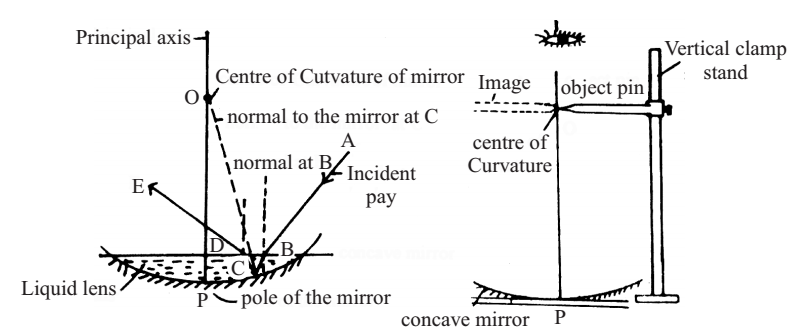# Comparision of Refractive Indices Experiment

Experiment: To compare the refractive indices of two transparent liquids using a concave mirror and a single pin.

When the object is placed on the centre of curvature of the concave mirror, the real inverted image is formed at the centre of curvature (i.e. at the same point).

When a ray of light falls normally on the concave mirror, it retraces its path backwards along the same line.

Refractive index of a liquid = Real radius of curvature of a mirror / Its apparent radius of curvature after filling its cavity with the transparent liquid

Material Required

A vertical clamp stand, plumb line and a metre scale, a pin, a concave mirror and the experimental liquids

### How To Set Up

1. Put the concave mirror on a horizontal plateform (Table).

2. Put the vertical clamp stand near the concave mirror and clamp the object pin horizontally in the vertical rod of the stand. Adjust the tip of the object pin just vertically above the centre of the concave mirror.### How To Perform Experiment

1. Fix the object pin in some position on the vertical stand sufficiently above the concave mirror.

2. Put your one eye vertically above the pin looking for the real inverted image of the pin in the concave mirror.

3. Move your eye slightly on to either side to find the parallax between the object pin and its real image.

4. Move the object pin on the vertical stand to a higher or lower position so as to coincide it with the image position. Now adjust carefully to remove the parallax between the object pin and its image. This makes the height of the image and the object pin above the concave mirror to be the same.

5. Measure, the height of the tip of the object pin from the pole (centre) of the concave mirror with the help of a plumb line and metre scale. This gives you h1 = real radius of curvature of the concave mirror.

6. Now fill the hollow cavity of the concave mirror with the given transparent experimental liquid (say, water). Wait for a minute so that the liquid becomes stationary and its level horizontal.

7. Again look for the image of the object pin in the concave mirror containing liquid. The image now appears to be formed nearer to the conave mirror than the object pin. Move the object pin on the vertical stand downwards towards the mirror to again coincide the image and the object pin. Remove the parallax between the two as before.

This gives you h2 = apparent radius of curvature of the combination pf concave mirror and the liquid lens which is equal to the distance between the object pin and the pole of the concave mirror. Measure this.

8. Repeat the procedure thrice and calculate the mean value of h1 and h2.

9. Now, calculate the refractive index (n) of the liquid using the formula

n = h1/h2

10. Repeat the observation for h2 with another transparent liquid and compare their refractive indices.

11. Find out percentage error in your results:

The standard value of Refractive Index of liquid = x (from the book)

Measured value = n

Error = n - x

Percentage Error = (n - x)/x × 100

### Sources of Error

1. If the tip of the object pin is not touching the tip of its image while removing the parallax between them, there may be difficulty in judging the correct position of non-parallax. The error may also arise in the values of h1 and h3 if the object pin is not horizontal.

2. The distances h1 and h2, are measured from the pole of the mirror to the tip of the object pin. However, when transparent liquid is filled in the cavity of the concave mirror, the pole gets slightly shifted upwards which cause an error in the measurement of h2 and hence in the result.

3. If the platform, on which the concave mirror is placed is not exactly horizontal, its principal axis will not be vertical but slightly oblique. This will lead to errors in the values of h1 and h2 measured vertically.